变量系列教材 （三）- 什么是Java的字面值 变量系列教材 （五）- Java的命名规则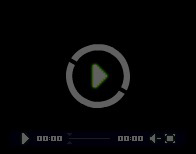8分33秒

## 转换规则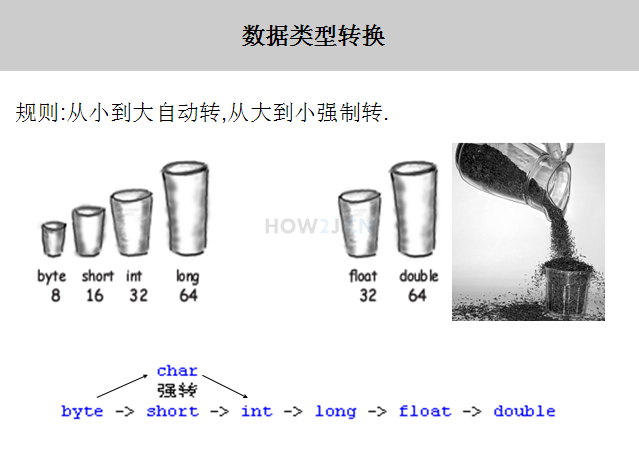public class HelloWorld { public static void main(String[] args) { char c = 'A'; short s = 80; //虽然short和char都是16位的，长度是一样的 //但是彼此之间，依然需要进行强制转换 c = (char) s; //直接进行转换，会出现编译错误 s = c; } }
```public class HelloWorld {

public static void main(String[] args) {

char c = 'A';
short s = 80;

//虽然short和char都是16位的，长度是一样的
//但是彼此之间，依然需要进行强制转换
c = (char) s;
//直接进行转换，会出现编译错误
s = c;

}
}
```

## 低精度向高精度转换

long l = 50;
int i = 50;

l 是long类型的，其类型长度是64位
i 是int类型的，其类型长度是32位

l = i;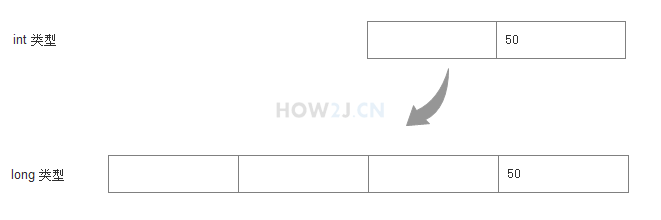public class HelloWorld { public static void main(String[] args) { long l = 50; int i = 50; //int比较小，要放进比较大的long,随便怎么样，都放的进去 l = i; } }
```public class HelloWorld {

public static void main(String[] args) {

long l = 50;
int i = 50;

//int比较小，要放进比较大的long,随便怎么样，都放的进去
l = i;

}
}
```

## 高精度向低精度转换

byte b = 5;
int i1 = 10;
int i2 = 300;
b = i1;
b=i2;

b的类型是byte,其长度是8，最大只能放127
i1 的类型是int, 其长度是32,最大，反正就是很大了，超过127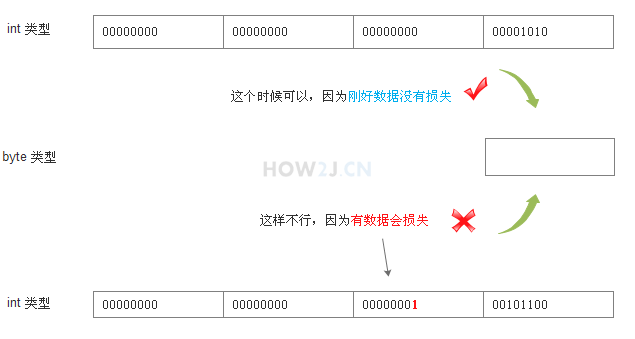public class HelloWorld { public static void main(String[] args) { byte b = 5; int i1 = 10; int i2 = 300; b = (byte) i1; //因为i1的值是在byte范围之内，所以即便进行强制转换 //最后得到的值，也是10 System.out.println(b); //因为i2的值是在byte范围之外，所以就会按照byte的长度进行截取 //i2的值是300，其对应的二进制数是 100101100 //按照byte的长度8位进行截取后，其值为 00101100 即44 b =(byte) i2; System.out.println(b); //查看一个整数对应的二进制的方法： System.out.println(Integer.toBinaryString(i2)); } }

## 练习-类型转换

姿势不对,事倍功半! 点击查看做练习的正确姿势
short a = 1;
short b = 2;

## 答案-类型转换1分14秒 本视频采用html5方式播放，如无法正常播放，请将浏览器升级至最新版本，推荐火狐，chrome，360浏览器。 如果装有迅雷，播放视频呈现直接下载状态，请调整 迅雷系统设置-基本设置-启动-监视全部浏览器 (去掉这个选项)。 chrome 的 视频下载插件会影响播放，如 IDM 等，请关闭或者切换其他浏览器

public class HelloWorld { public void method1(final int j) { short a = 1; short b = 2; short c = (short) (a+b); } }
```public class HelloWorld {

public void method1(final int j) {
short a = 1;
short b = 2;
short c = (short) (a+b);
}

}
```

 变量系列教材 （三）- 什么是Java的字面值 变量系列教材 （五）- Java的命名规则

HOW2J公众号，关注后实时获知最新的教程和优惠活动，谢谢。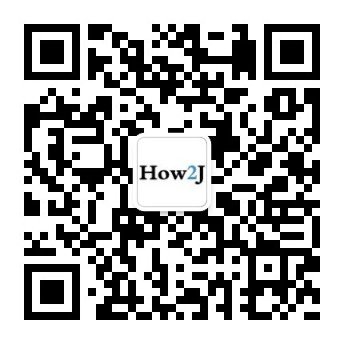2022-04-25 int转换为低精度为什么用int型变量转低精度报错但int型数值转就没问题
 努力学习-早日上岸 关于 JAVA 基础-变量-类型转换 的提问 int decVal = 26; byte d; d=26; 这样没问题，但是 int decVal = 26; byte d; d=decVal; 这样写就会报错不能将int转换为byte型4 个答案

cy20000529

public class bian { public static void main(String[] args){ short a = 1; short b = 2; short c = (short) (a+b); System.out.println(c); }

A安1_

A安1_

xiaoba_java

d=26是bety型变量可以接收符合bety型范围的数据 而d=decVal，d是bety型变量，decVal是int型变量，int型变量不能直接转成bety型变量，需要强制转换d=（bety）decVal；

2021-08-09 答案视频没声音
 liyaoguang 关于 JAVA 基础-变量-类型转换 的提问 答案视频在后半段的时候突然没有声音了，请更换一下正确的录制视频。

7 个答案

0805_3

public class TypeConversion { public static void main(String[] args) { short a = 1; short b = 2; short c = (short) (a + b); System.out.println(c); } }

fyc7015656

public class bian { public static void main(String[] args){ short a = 1; short b = 2; short c = (short) (a+b); System.out.println(c); }

yolanda_hello_java

@hefutong 我把你的方法复制到vs code里，提示了多种语法错误，想问一下，你这边的代码，是复制的时候格式变了，还是什么情况， 你的这种方法用的是哪一个指令？这个指令的语法规则又是怎么用的？

public static void main(String[] args) { short a = 1; short b = 2; short c=(short)(a+b); System.out.println("a+b是什么数据类型？"); System.out.println(c); }

hefutong

short a = 1; short b = 2; System.out.println(getType(a+b)); } //获取变量类型的方法 private static String getType(Object o) { return o.getClass().toString(); }
`输出int型`

happar886

```short a=1;
//        a=a+1;  类型不匹配，不能从int转换为short
a= (short) (a+1);//第一种方法
a+=1;//第二种方法```

```byte =c;
short a =1;
short b =2;
c =(byte)(a+b);
System.out.println(c);```

2021-07-31 我如何知道运算结果是多少
2021-07-04 高低精度转换
2020-12-03 1或2 不属于short类型吗？